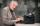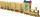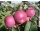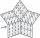# Honza

Honza is 13 years old and Peter 21 years old. After how many years are their ages will be at ratio 7: 9?

Result

x =  15

#### Solution:

9*(13+x) = 7*(21+x)

2x = 30

x = 15

Calculated by our simple equation calculator.

Leave us a comment of example and its solution (i.e. if it is still somewhat unclear...):

Showing 0 comments:Be the first to comment!#### To solve this example are needed these knowledge from mathematics:

Need help calculate sum, simplify or multiply fractions? Try our fraction calculator. Do you have a linear equation or system of equations and looking for its solution? Or do you have quadratic equation?

## Next similar examples:

1. Six yearsIn six years Jan will be twice as old as he was six years ago. How old is he?
2. MidnightHow many hours are, if the time that elapsed since 8:00 is 2/5 of the time that will past till midnight?
3. Three monksThree medieval monks has task to copy 600 pages of the Bible. One rewrites in three days 1 page, second in 2 days 3 pages and a third in 4 days 2 sides. Calculate for how many days and what day the monks will have copied whole Bible when they begin Wednesd
4. Two typistsThere are two typists who are rewriting the material 814 pages. First can it handle rewrite yourself for 24 days; the second 12 days. First typist wrote material yourself 4 days rest rewrites yourself second typist. How many days will it take rewriting alt
5. SchoolSchool attend 792 children, boys and girls ratio is 4:5. How many more girls go to school (than boys)?
6. Boys to girlsThe ratio of boys to girls in a party is 3:5 . If 6 more boys arrived and 4 girls left the party, the ratio of boys to girls would be 5:6 . How many are in the party originally?
7. Mason with assistantMason had to complete the work in 10 days. Two and a half days later his assistant arrived. Together they completed the work in next three and a half days. How many days would he need an assistant for the same work?
8. ThereThere are 32 trees of three species in the orchard. 25% cherries, three eighty pears, others plums. How many plums are in the orchard?
9. Three peopleThree people start doing a work at a same time. The first worked only 2 hours. The second ended 3 hours before the end. On an individual basis, it would take the first time to do the work 10 hours, second 12 hours and 15 hours third. How many hours did it.
10. Ratio 11Simplify this ratio 10 : 1/4
11. Percentage 1052 shorts and missed 13. Calculate percentage
12. Unknown number 23Find 2/3 of unknown number, which is two thirds of the 99.
13. Simple equation 6Solve equation with one variable: X/2+X/3+X/4=X+4
14. EqnSolve equation with fractions: 2x/3-50=40+x/4
15. Equation 20In given equation: 8/9-4/5=2/9+x, find x
16. Fifth of the numberThe fifth of the number is by 24 less than that number. What is the number?
17. NovakNovak needed to dig up three of the same pit in the garden. The first pit dug father alone for 15 hours. His second dig son helped him and it did that in six hours. The third pit dug son himself. How long it took him?